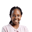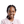Trusted answers to developer questions
Trusted Answers to Developer Questions

Related Tags

hyperparameters
parameters

# What is the difference between parameters and hyperparameters?Joy Kareko

Grokking Modern System Design Interview for Engineers & Managers

Ace your System Design Interview and take your career to the next level. Learn to handle the design of applications like Netflix, Quora, Facebook, Uber, and many more in a 45-min interview. Learn the RESHADED framework for architecting web-scale applications by determining requirements, constraints, and assumptions before diving into a step-by-step design process.

### Introduction

Machine learning is a field of mathematics where computers use predefined sets of instructions to learn or find patterns in data. These predefined sets of instructions that instruct how a computer will solve a problem or accomplish a task are called algorithms.

There are many types of algorithms in machine learning that function differently depending on the problem that needs to be solved or the task that needs to be accomplished.

Once an algorithm is used to find patterns in data, it’s referred to as a model. Models consist of parameters and hyperparameters.

We shall be discussing the differences between parameters and hyperparameters in this shot.

Diagram showing the model training sequence

### Parameters vs. hyperparameters

Parameters are internal to a model and are estimated from the data itself.

Once a model’s parameters are determined, the model can be used to make predictions on unseen data.

For example, in a linear regression model, we have independent variables/features and one dependent variable called the target. This is illustrated in the equation below:

Y = a + bX


Here, Y is the target and X is the independent variable. In addition, b is the coefficient of X and a is the intercept or value of Y when X is zero.

The independent variable is used to predict the target feature.

In such a case, we use a linear regression algorithm to learn the underlying patterns in the data. Once we train the model, we derive coefficients from the independent features:

Y = 20 + 3X


The coefficient 3 and 20 in this model are the parameters. These parameters are then used to obtain predictions on data.

Hyperparameters, on the other hand, are external to the model. They can be configured or tuned manually to maximize a model’s performance. They come with defaults and are set before the modeling starts. This is done to optimize the model.

In the example above on the linear regression model, there are no definite hyperparameters to tune. However, variants of the linear regression algorithms, such as ridge regression and lasso regression, use regularization to obtain the best model’s parameters.

Another example of a hyperparameter would be k in the k-nearest neighbors (KNN) algorithm, where tuning helps obtain the optimal number of clusters, although the default value is 5.

RELATED TAGS

hyperparameters
parameters

CONTRIBUTORJoy Kareko

Grokking Modern System Design Interview for Engineers & Managers

Ace your System Design Interview and take your career to the next level. Learn to handle the design of applications like Netflix, Quora, Facebook, Uber, and many more in a 45-min interview. Learn the RESHADED framework for architecting web-scale applications by determining requirements, constraints, and assumptions before diving into a step-by-step design process.

Keep Exploring

Learn in-demand tech skills in half the time